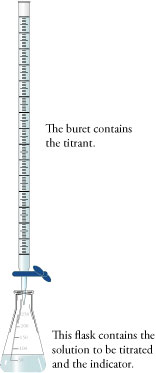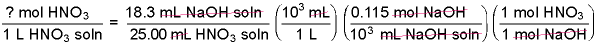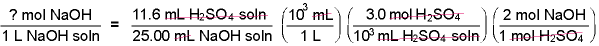﻿ Titration Problems

#Titration Problems

• Molarities of acidic and basic solutions are often used to convert back and forth between moles of solutes and volumes of their solutions, but how were the molarities of these solutions determined? This webpage describes a procedure called titration, which can be used to find the molarity of a solution of an acid or a base.

In titration, one solution (solution 1) is added to another solution (solution 2) until a chemical reaction between the components in the solutions has run to completion. Solution 1 is called the titrant, and we say that it is used to titrate solution 2. The completion of the reaction is usually shown by a change of color caused by a substance called an indicator.

A typical titration proceeds in the following way. A specific volume of the solution to be titrated (solution 2) is poured into an Erlenmeyer flask (Figure 1). For example, 25.00 mL of a nitric acid solution of unknown concentration might be added to a 250 mL Erlenmeyer flask.

A solution of a substance that reacts with the solute in solution 2 is added to a buret. (A buret is a laboratory instrument used to add measured volumes of solutions to other containers.) This solution in the buret, which has a known concentration, is the titrant. The buret is set up over the Erlenmeyer flask so the titrant can be added in a controlled manner to the solution to be titrated (Figure 1). For example, a 0.115 M NaOH solution might be added to a buret, which is set up over the Erlenmeyer flask containing the nitric acid solution.Figure 1    Setup for a Typical Titration     In a typical titration, the titrant in the buret is added to the solution in the Erlenmeyer flask until the indicator changes color to show that the reaction is complete.

An indicator is added to the solution being titrated. The indicator is a substance that changes color when the reaction is complete. In our example, phenolphthalein, which is a commonly used acid‑base indicator, is added to the nitric acid solution in the Erlenmeyer flask. Phenolphthalein has two chemical forms. In acidic conditions, it is in the acid form, which is colorless. In basic conditions, an H+ ion is removed from each phenolphthalein molecule, converting it to its base form, which is red.

The titrant is slowly added to the solution being titrated until the indicator changes color, showing that the reaction is complete. This stage in the procedure is called the endpoint. In our example, the NaOH solution is slowly added from the buret until the mixture in the Erlenmeyer flask changes from colorless to red. The OH ions in the NaOH solution react with the H3O+ ions in the HNO3 solution.

H3O+(aq)  +  OH(aq)    H2O(l)

As long as there are excess H3O+ ions in the solution, the solution stays acidic, the phenolphthalein stays mostly in the acid form, and the solution is colorless. When enough NaOH solution is added to react with all of the H3O+ ions, the reaction is complete. When a small amount of extra NaOH solution is added, perhaps one drop, there will be an excess of hydroxide ions, OH, in solution. These react with the phenolphthalein molecules, changing them from the acid form to the base form. Because the base form is red, the solution turns red, telling us that the reaction is complete (or just slightly beyond complete).

The volume of titrant added from the buret is measured. For our example, let's assume that 18.3 mL of 0.115 M NaOH has been added. The following setup shows how the molarity of the nitric acid solution can be calculated from this data.0.0842 M HNO3

The first step the unit analysis thought-process is to clearly identify the units that you want. Molarity describes the number of moles of solute per liter of solution, so we start by placing moles of HNO3 over 1 L HNO3 solution.

Because molarity is a ratio of two units, we begin our calculation with a ratio of two units. Knowing that we want volume of HNO3 solution on the bottom when we are done, we place 25.00 mL HNO3 solution on the bottom at the start. We place 18.3 mL NaOH solution on the top of our ratio, giving us the ratio of two units overall that we want.

We convert milliliters of HNO3 solution to liters of HNO3 solution using the relationship between milliliters and liters. The last two conversion factors convert from amount of one substance in a chemical reaction (mL NaOH solution) to amount of another substance in the reaction (mol HNO3). Thus this is an equation stoichiometry problem that requires at its core the conversion of moles of NaOH to moles of HNO3 using the molar ratio for the reaction between them.

NaOH(aq)  +  HNO3(aq)    NaNO3(aq)  +  H2O(l)

In order to use the molar ratio to convert from moles of NaOH to moles of HNO3, we need to convert from volume of NaOH solution to moles of NaOH using the molarity as a conversion factor.

Sample Study Sheet: Acid-Base Titration Problems

Tip-off – You are given the volume of a solution of an acid or base (the titrant – solution 1) necessary to react completely with a given volume of solution being titrated (solution 2). You are also given the molarity of the titrant (solution 1). You are asked to calculate the molarity of solution 2.

General Procedure

• Use the unit analysis process, with the following general format.The first conversion factor is used only when you are not given liters of solution 2. (Because you are usually given milliliters, you may instead need to use a conversion factor that converts from milliliters to liters.)

The second conversion factor is used only when you are not given either milliliters or liters of solution 1. (You are usually given milliliters, so if your molarity conversion factor is in the form that includes "103 mL #1 soln", this conversion factor is not necessary.)

The coefficients in the final conversion factor come from the balanced equation for the reaction.

Complete the calculation in the usual way.

EXAMPLE:  Calculating Molarity from Titration Data

Titration reveals that 11.6 mL of 3.0 M sulfuric acid are required to neutralize the sodium hydroxide in 25.00 mL of NaOH solution. What is the molarity of the NaOH solution?

Solution:

H2SO4(aq)  +  2NaOH(aq)    →   2H2O(l)  +  Na2SO4(aq)=  2.8 M NaOH

Chiral Publishing Company